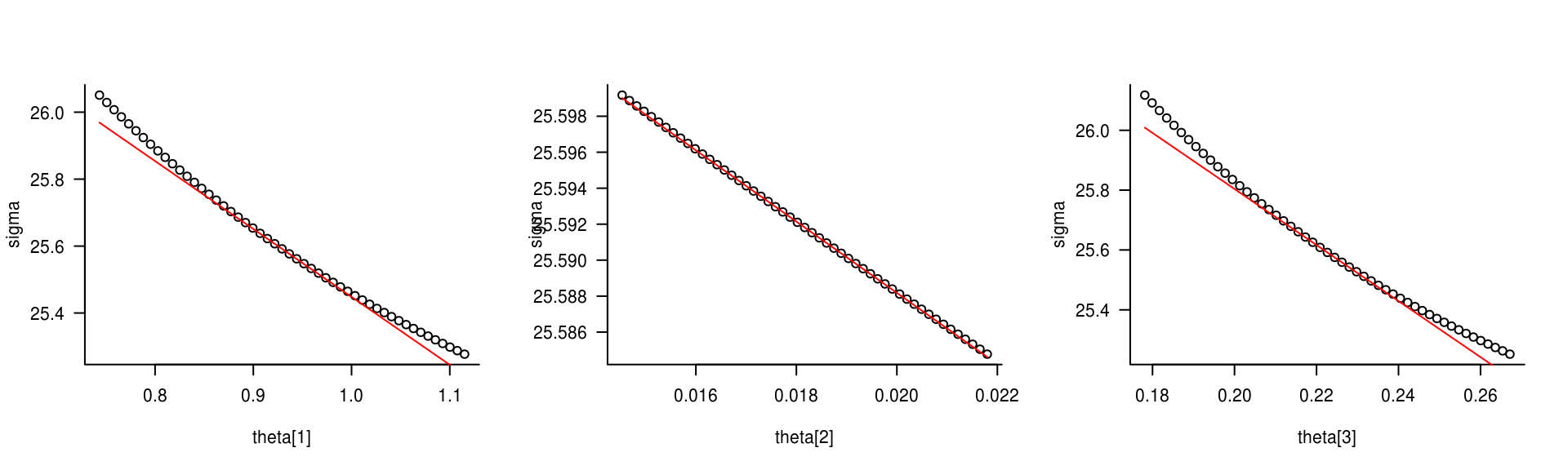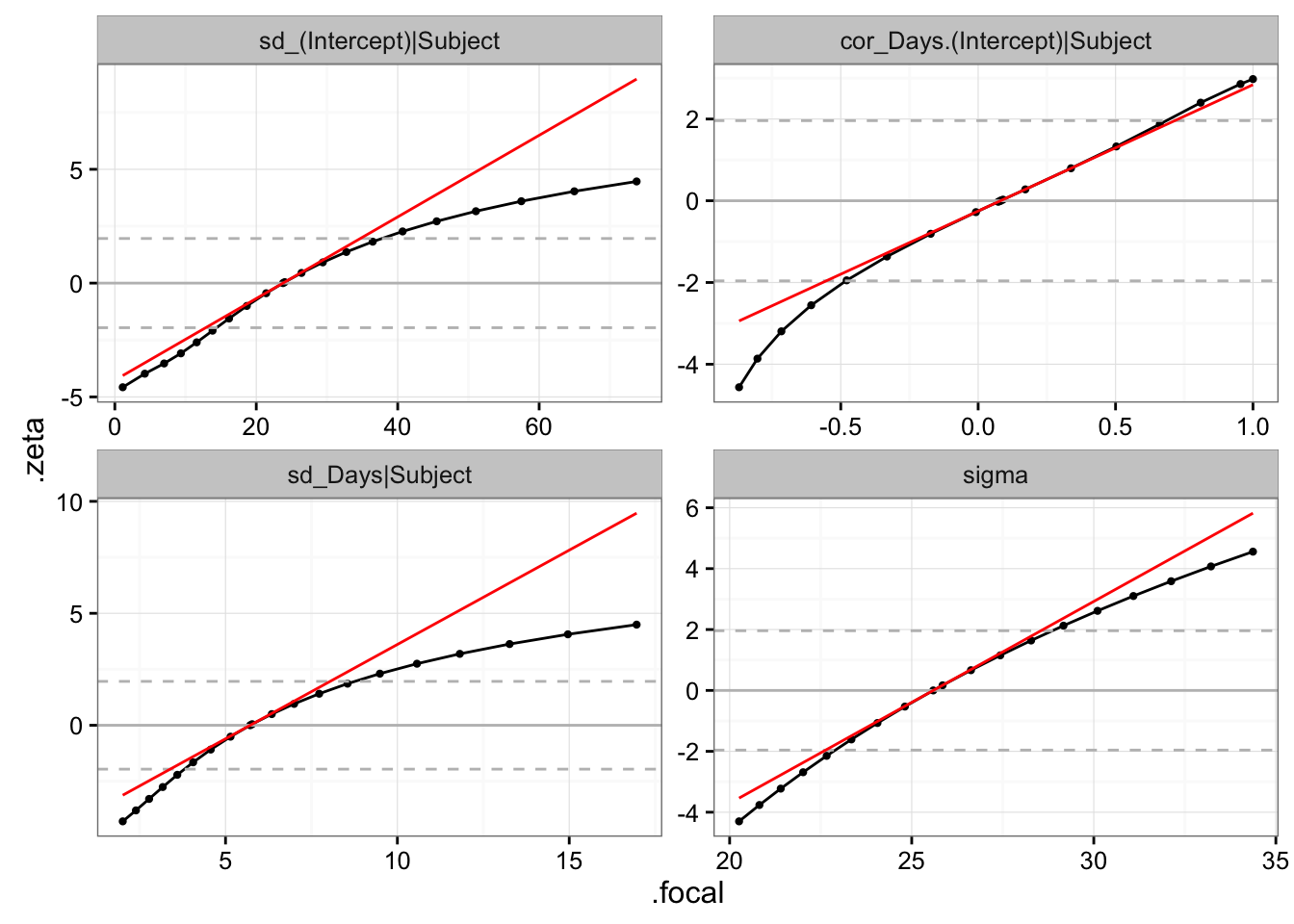## Introduction

lme4 users frequently request the capability to compute the variances (standard errors, variance-covariance matrix) of the random-effects variance parameters. lme4 has switched to providing profile and parametric bootstrap, but not Wald confidence intervals on the random-effect variances, because the Wald intervals on variance-covariance parameters are often really bad. Nevertheless, one sometimes does want the asymptotic (Wald) variance-covariance matrix of the variance-covariance parameters … This document shows how to do that for merMod objects, and discusses the issues more generally.

In the nlme package the Wald variances are (implicitly) available via the intervals() function in the nlme package, which returns confidence intervals based on Wald intervals on the transformed (“unconstrained” scale, i.e. the log scale for variance parameters and the (tanh(x)/2) scale for correlation parameters; the underlying approximate variance-covariance matrices (on the unconstrained scale) are stored as the $apVar component in the model object. The basic problem is that lme4’s deviance function uses a scaled Cholesky-factor parameterization, e.g. for a random slope model the variance-covariance matrix is $\Sigma = \sigma^2 L L^T = \sigma^2 \left( \begin{array}{cc} \theta_1 & 0 \\ \theta_2 & \theta_3 \end{array} \right) \left( \begin{array}{cc} \theta_1 & \theta_2 \\ 0 & \theta_3 \end{array} \right)$ It’s easy enough to get an approximate Hessian of the $$\theta$$ parameters by finite differences, but not so easy to get estimates of the variance-covariance parameters. There are a few strategies for converting from this to the variance scale: • Use an internal deviance function (devfun2) that is parameterized on the $$\sigma$$ scale. (At present devfun2 only works for ML, not REML; this could be hacked …) • Use the delta method on the equation above (but we need the variance in the $$\sigma^2$$ parameter … ?) • Compute dependence of the $$\sigma$$-scale parameters on the $$\theta$$ parameters and use the delta method. ## Preliminaries library("lme4") library("numDeriv") library("ggplot2"); theme_set(theme_bw()) Fit the good old sleepstudy model: fm1 <- lmer(Reaction ~ Days + (Days | Subject), sleepstudy) For comparison with the devfun2 likelihood profile (which uses ML rather than REML) we also fit the ML equivalent: fm1.ML <- refitML(fm1) Also fit with lme so that we can compare the results of intervals(): library(nlme) fm1.lme <- lme(Reaction ~ Days, random = ~Days| Subject, sleepstudy) fm1.lme.ML <- update(fm1.lme,method="ML") ## Delta method Suppose we have parameters $$\mathbf \theta$$ (possibly also including fixed-effect parameters in this case; I mean the full set of parameters that determine the deviance), and a variance-covariance matrix $$\Sigma_{\mathbf \theta}$$ for them, but we have a set of derived parameters $$\mathbf \sigma$$ and we want to get $$\Sigma_{\mathbf \sigma}$$. Then all we actually need is the Jacobian matrix $${\mathbf J} = ( \partial \sigma_i/\partial \theta_j )$$, which we can get by finite differencing; then $$\mathbf J^T \Sigma_{\mathbf \theta} \mathbf J$$ should give us $$\Sigma_{\mathbf \sigma}$$. In particular: ## generate a model with a modified set of theta parameters update.lmerMod <- function(object,theta,...) { if (missing(theta)) return(update.default(object,...)) object2 <- object ## deep-copy the (only) reference-class slots ... object2@pp <- object@pp$copy()
object2@resp <- object@resp$copy() object2@pp$setTheta(theta)
dd <- as.function(object2)
dval <- dd(theta)  ## update internal structures
## object2@resp$updateMu(object2@resp$mu)  ## ?? not helping/not necessary

mkMerMod(environment(dd),
opt=list(par=theta,fval=dval,conv=0),
fr=model.frame(object2),
reTrms=getME(object2,
c("Zt","Lambdat","Lind","theta",
"lower","flist","cnms","Gp"))
)
}
## for GLMMs: update.glmerMod will need a beta parameter;
## object@pp$setBeta0(beta) ## dd <- update(object,devFunOnly=TRUE) ## parvec <- c(theta,beta) ## dval <- dd(parvec) ## opt=list(par=parvec,...) ## test: is it safe? fm2 <- update(fm1,theta=c(2,2,2)) orig_theta <- getME(fm1,"theta") stopifnot(identical(unname(getME(fm2,"theta")),c(2,2,2))) stopifnot(identical(orig_theta,getME(fm1,"theta"))) tn <- function(object) { c(names(getME(object,"theta")),"sigma") } ## compute standard deviations/correlations for a given theta vector outfun <- function(object,t) { newmod <- update(object,theta=t) av <- as.data.frame(VarCorr(newmod), order="lower.tri") r <- setNames(av[,"sdcor"],tn(object)) return(r) } Test derivative calculations for $$\sigma$$: pp <- getME(fm1.ML,"theta") Jmat <- jacobian(outfun,pp,object=fm1.ML) slicefun <- function(pos) { tvec <- seq(0.8*pp[pos],1.2*pp[pos],length=51) svec <- sapply(tvec,function(x) { pp2 <- pp pp2[pos] <- x outfun(fm1.ML,t=pp2)["sigma"] }) list(x=tvec,y=svec) } ss <- lapply(1:3,slicefun) par(las=1,bty="l",mfrow=c(1,3)) for (i in 1:3) { plot(ss[[i]],xlab=paste0("theta[",i,"]"),ylab="sigma") curve((x-pp[i])*Jmat[4,i]+sigma(fm1.ML),add=TRUE,col=2) }Does my update function correctly update sigma? Apparently so … up1 <- update(fm1,theta=c(2,2,2)) ## update 'from scratch' up2 <- suppressWarnings(update(fm1, start=c(2,2,2), control=lmerControl(optCtrl=list(maxfun=1)))) identical(unname(getME(up2,"theta")),c(2,2,2)) ##  TRUE all.equal(sigma(up1),sigma(up2)) ##  TRUE waldVar1 <- function(object) { pp <- getME(object,"theta") ## GLMM: unlist(getME(dd,c("theta","beta"))) Jmat <- jacobian(outfun,pp,object=object) dimnames(Jmat) <- list(tn(object),paste0("theta",seq(ncol(Jmat)))) dd <- as.function(object) ## deviance/REMLcrit function hh <- 1/2*hessian(dd,pp) ## ... calculate information matrix ... ## 1/2 = deviance to log-lik scale) ## vv <- solve(hh) ## invert to get Wald vars of theta parameters ## m2 <- Jmat %*% vv %*% t(Jmat) ## delta method ## slightly better linear algebra: m1 <- Jmat %*% solve(hh,t(Jmat)) return(m1) } wsd1 <- sqrt(diag(waldVar1(fm1))) wsd1.ML <- sqrt(diag(waldVar1(fm1.ML))) As we’ll see, the estimate of the standard deviation for sigma is off by a factor of about 2 (but not exactly) compared to both the second method (using devfun2) and the lme results. I can’t figure out why right now - this would occur naturally if I got confused between variance and standard deviation scale, but (a) it should be an exact factor of 2 (b) if so I can’t find where it’s happening. ### Finite differences on the $$\sigma$$ scale The other way to do this is using the internal devfun2() function, which is used within profile — it is essentially the same as the basic devfun(), but it uses a standard deviation/correlation parameterization (and adds the residual standard deviation as a parameter) rather than a Cholesky parameterization: waldVar2 <- function(object) { ## test for/warn if ML fit? dd <- lme4:::devfun2(object,useSc=TRUE,signames=FALSE) nvp <- length(attr(dd,"thopt"))+1 ## variance parameters (+1 for sigma) pars <- attr(dd,"optimum")[seq(nvp)] ## var params come first hh <- hessian(dd,pars) ## factor of 2: deviance -> negative log-likelihood vv <- 2*solve(hh) nn <- tn(object) dimnames(vv) <- list(nn,nn) return(vv) } ## would be identical applied to fm1 (would get ML-ized anyway) wsd2.ML <- sqrt(diag(waldVar2(fm1.ML))) At the moment devfun2() cannot be used for REML fits (as it is designed for computing likelihood profiles, that wouldn’t make much sense). ### Convert scales I have a bunch of code now (not shown here) for converting estimates+standard deviations to confidence intervals on a different scale. We translate the estimates and standard deviations to the link scale; compute the Wald confidence intervals on that scale; and back-transform. ### Results and comparisons Now that we have the Wald variance-covariance matrix, I’m going to cook up a little bit of structure that will let us use confint.default to compute the Wald intervals: pars <- as.data.frame(VarCorr(fm1.ML),order="lower.tri")[,"sdcor"] names(pars) <- tn(fm1.ML) vhack1 <- list(coefficients=pars,vcov=waldVar1(fm1.ML)) vhack2 <- list(coefficients=pars,vcov=waldVar2(fm1.ML)) vcov.default <- function(object,...) object$vcov
## convert variance parameters to log scale,
## correlation to atanh scale (to match lme)
pars=list(c(1,3,4),2))
(wc1S <- confint2(vhack1,trans=trans_list1))
##                              2.5 %     97.5 %
## Subject.(Intercept)      15.194690 37.2179646
## Subject.Days.(Intercept) -0.566403  0.7472092
## Subject.Days              3.856526  8.4745162
## sigma                    24.303564 26.9483530
(wc2S <- confint2(vhack2,trans=trans_list1))
##                               2.5 %     97.5 %
## Subject.(Intercept)      15.0170918 37.6581199
## Subject.Days.(Intercept) -0.5664029  0.7472091
## Subject.Days              3.8054678  8.5882203
## sigma                    22.8004578 28.7249069
## non-scaled versions for comparison
wc1 <- confint2(vhack1)
wc2 <- confint2(vhack2)
(lmeint <- intervals(fm1.lme.ML,which="var-cov"))
## Approximate 95% confidence intervals
##
##  Random Effects:
##   Level: Subject
##                            lower       est.     upper
## sd((Intercept))       15.0466764 23.7803757 37.583467
## sd(Days)               3.8120855  5.7168073  8.573230
## cor((Intercept),Days) -0.4743728  0.0813321  0.590688
##
##  Within-group standard error:
##    lower     est.    upper
## 22.80225 25.59184 28.72270

#### Comparison with likelihood profile

Compute the likelihood profile:

## automatically ML-ified
pp <- profile(fm1,which="theta_",signames=FALSE)

The linear approximation to the profile for a focal parameter $$x$$ should be $$\zeta \approx (x-\hat x)/\sigma_x$$, where $$\hat x$$ is the MLE and $$\sigma_x$$ is the Wald standard deviation:

dfprof <- as.data.frame(pp)
mframe <- data.frame(.par=levels(dfprof\$.par),ctr=pars,slope=1/wsd2.ML)
dfprof <- merge(dfprof,mframe)
ggplot(dfprof,aes(.focal,.zeta))+geom_line()+geom_point(size=0.8)+
facet_wrap(~.par,scale="free")+
geom_hline(yintercept=0,color="gray")+
geom_hline(yintercept=c(-1.96,1.96),color="gray",lty=2)+
geom_line(aes(y=(.focal-ctr)*slope),color="red")In this particular example, there’s not a huge divergence from linearity over the range defined by the 95% confidence intervals (dashed horizontal lines) … as confirmed by the confidence intervals. (Should check with waldVar1, sort out problem with sigma …)